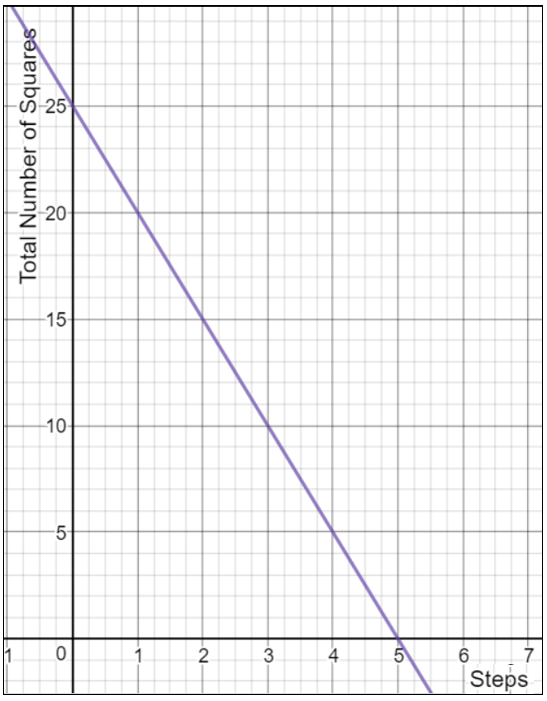# LINR 1 | Lesson 2 | Try This! | (Slope and $$y$$-Intercept Solutions)

1.  Johanna was saving her allowance to buy a bicycle that cost $225. She has$25 in her savings and intends to save an additional $15 per week. Slope = $$15$$ per week y-intercept = $$(0,25)$$ 2. The cost to prepare tacos can be represented by the linear equation $$c=\Large \frac{5}{4}\normalsize n+0.75$$, where $$c$$ represents the cost of making tacos, and $$n$$ represents the number of tacos made. Slope = $$\large\frac{5}{4}$$ dollars per taco or$1.25 per taco.

y-intercept = $$(0, 0.75)$$

3. The following is a graphical representation of the number of squares in each step of a linear growth pattern. Graph is created using Desmos.com.Slope = $$-5$$ squares per step

y-intercept = $$(0,25)$$

4. The table below represents the distance traveled (in miles) over time (in hours).

Time (hours) Distance Traveled (miles)
$$2$$ $$10$$
$$3$$ $$13.5$$
$$4$$  $$17$$
$$5$$ $$20.5$$

Slope = $$3.5$$ miles per hour

$$y$$-intercept = $$(0,3)$$